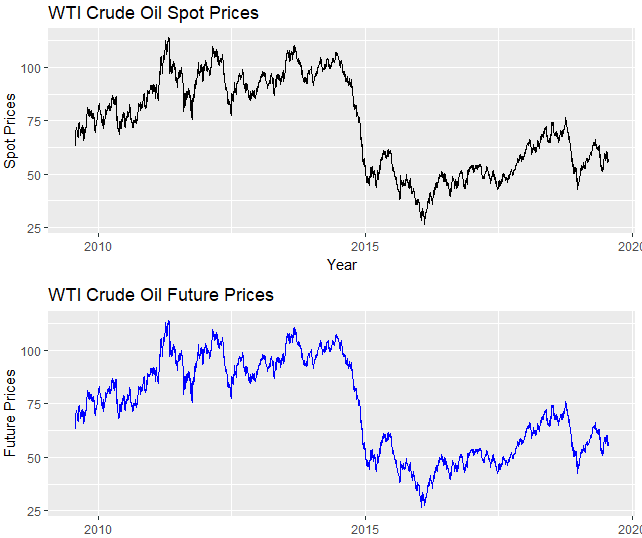# Comparing Futures vs Spot Prices for WTI Crude Oil

In this post, we will be comparing the Futures vs Spot Prices for WTI Crude Oil, i.e., the WTI Futures series with the WTI Spot series. The futures prices generally show high volatility and they are more volatile than the underlying spot price. For this purpose, it would be interesting to compare future and spot prices on the same asset and see some metrics of volatility. First we will plot both series for the same period and then will implement a function to get some volatility metrics for each series.

WTI Crude Oil Spot and Future PricesWTI Crude Oil Spot and Future Prices

The pattern for the two series is similar, but strictly it will be good to have volatility measures for both series. We will make an R function that compares series from spot and future prices WTI for the same period and then calculates the Interquartile Range (difference between 75th and 25th percentiles) and Standard Deviation for both  series. With this procedure we can compare volatility measures for the two series.

The future series is the one that we have been using along this tutorial which is the CME_CL_Data_ dataset and we will load oil spot data for WTI from a csv file. The function spotVsFutures will implement this task:

spotVsFutures <- function(file1,cl_future){

oilFuture <- cl_future
# Retrieve the first and last date from oilSpot dataframe
first_date <- oilSpot$date last_date <- tail(oilSpot$date,1)

# Subset the CME_CL_Data_ by the first date and last date of oilSpot data in order
# to have both series with same dates
CL <- subset(CME_CL_Data_,Date >= first_date & Date <= last_date)
# Calculate returns of both series
future_returns <- diff(log(CL$Last)) spot_returns <- diff(log(oil_spot$value))
# Make a dataframe with the returns of both series
df <- data.frame(cbind(spot_returns,future_returns))
# Obtain the standard deviation and the Interquartile Range for both returns series.
IQRSpot <- round(IQR(df$spot_returns),6) IQRFuture <- round(IQR(df$future_returns),6)
SDFuture <- round(sd(df$future_returns),6) SDSpot <- round(sd(df$spot_returns),6)
cat('Interquartile Range for spot and future returns of WTI are', c(IQRSpot,IQRFuture))
cat('\n')
cat('Standard Deviation for spot and future returns of WTI are', c(SDSpot,SDFuture))

}


We can now execute the function with our data.

file1 <- "C:/Users/Nicolas/Documents/Derivatives with R/OilSpot/wti-crude-oil-prices-10-year-daily-chart.csv"

spotVsFutures(file1,CME_CL_Data_)


By running the function we have the data that the Interquartile Range for the spot and future WTI Crude are 0.021679 and 0.022157 respectively. The Standard Deviations are 0.02039 and 0.0205. We conclude that for both metrics the WTI Future prices have higher values than the WTI spot prices.